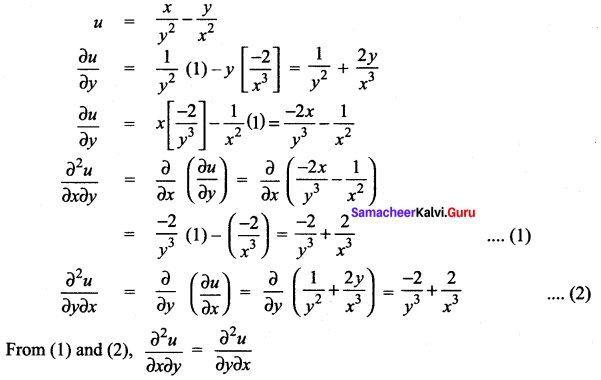# Samacheer Kalvi 12th Maths Solutions Chapter 8 Differentials and Partial Derivatives Ex 8.4

## Tamilnadu Samacheer Kalvi 12th Maths Solutions Chapter 8 Differentials and Partial Derivatives Ex 8.4

Question 1.
Find the partial derivatives of the following functions at the indicated points.
(i) f(x, y) = 3x2 – 2xy + y2 + 5x + 2, (2, -5)
(ii) g(x, y) = 3x2 + y2 + 5x + 2, (1, -2)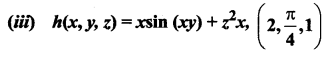(iv) G (x, y) = e*x + 3y log (x2 + y2), (-1, 1)
Solution: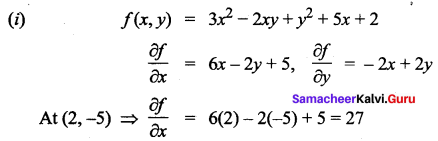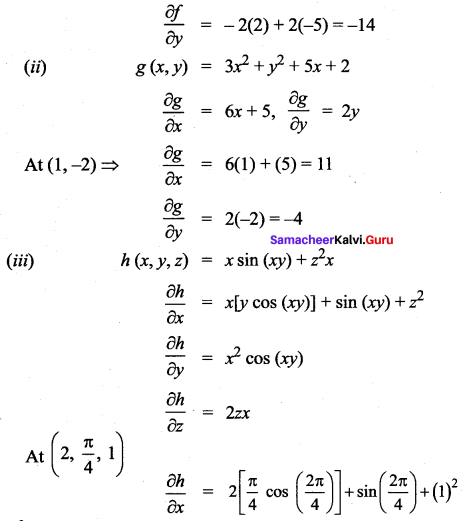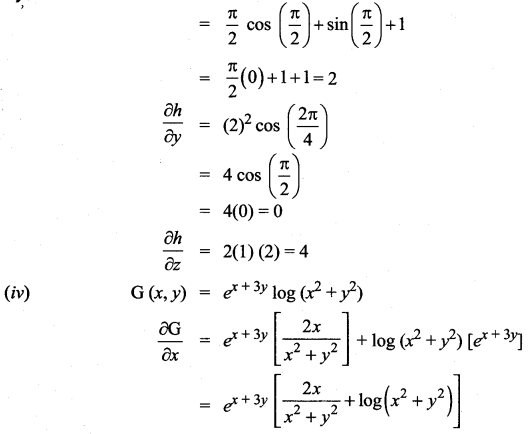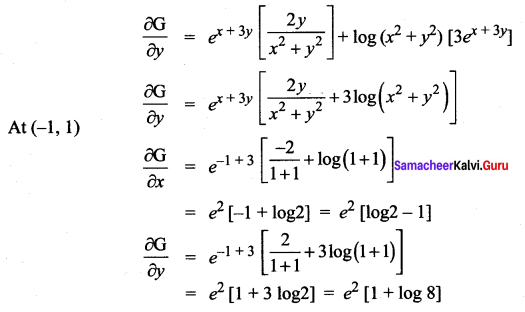Question 2.
For each of the following functions find the fx, fy and show that fxy = fyx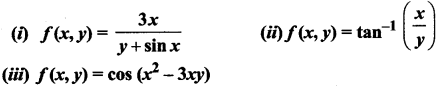Solution: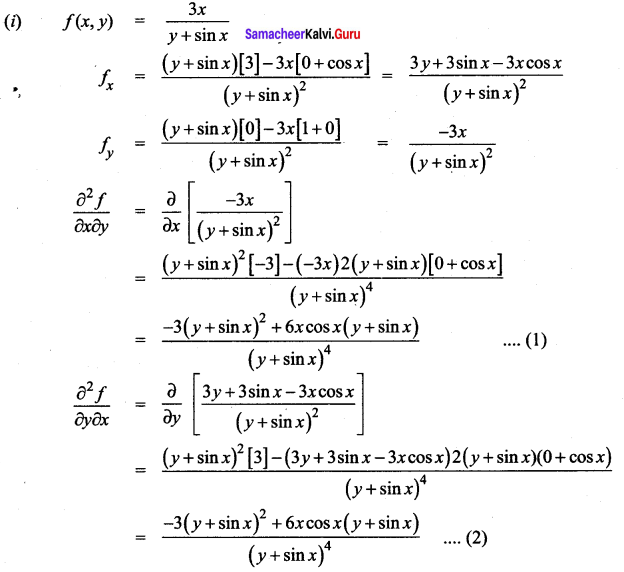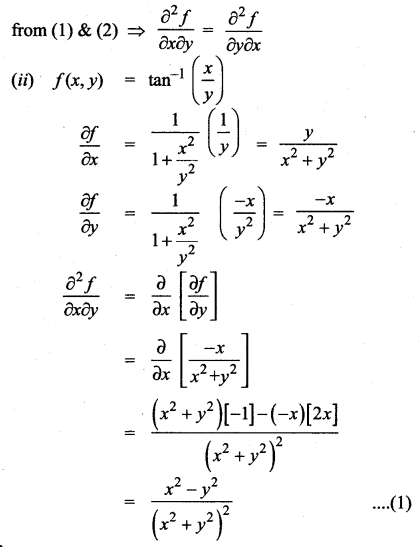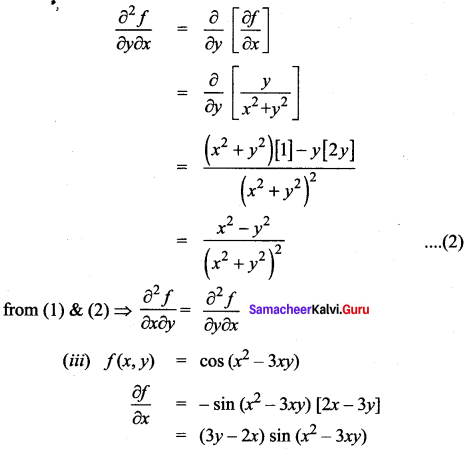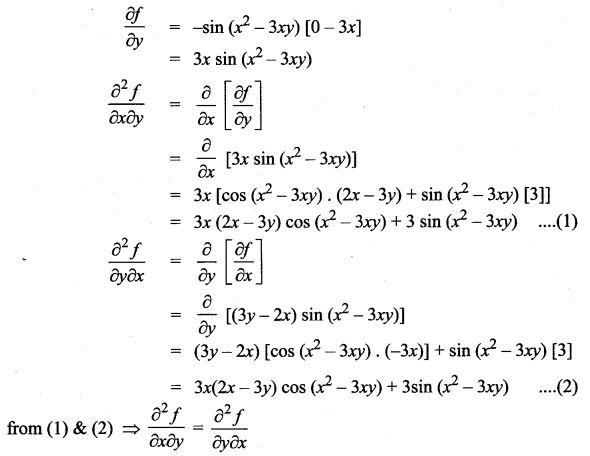Question 3.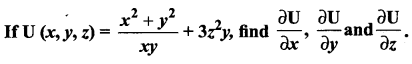Solution: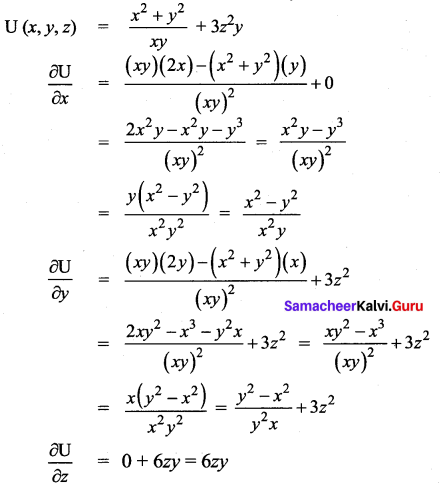Question 4.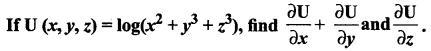Solution: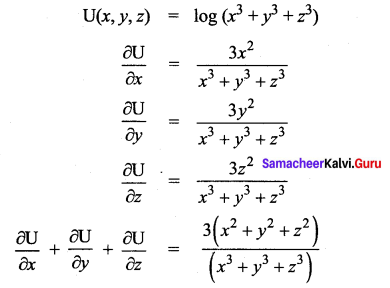Question 5.
For each of the following functions find the gxy, gxx, gyy and gyx.
(i) g(x, y) = xey + 3x2y
(ii) g(x, y) = log(5x + 3y)
(iii) g(x, y) = x2 + 3xy – 7y + cos(5x)
Solution: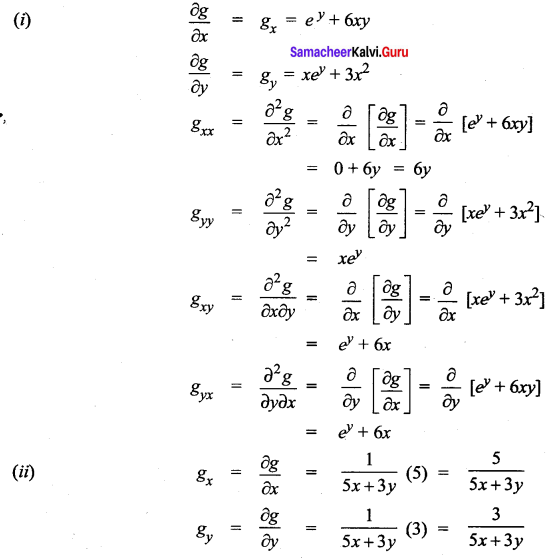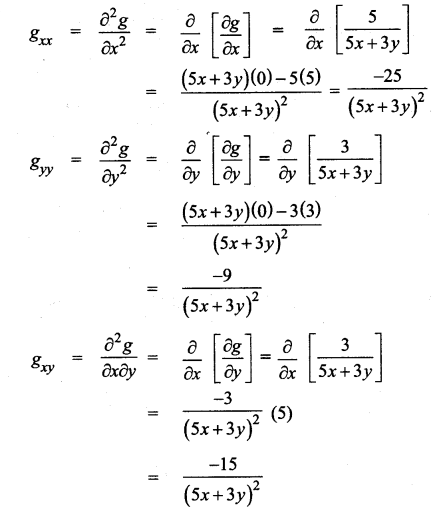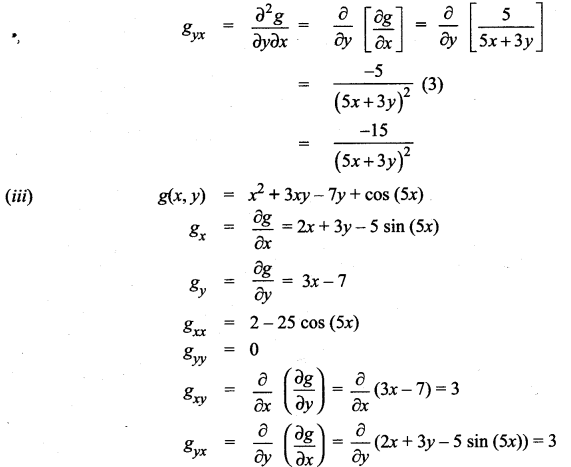Question 6.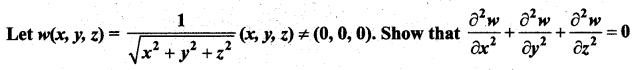Solution: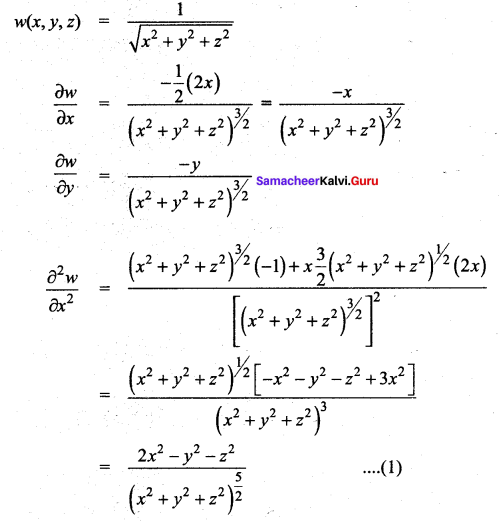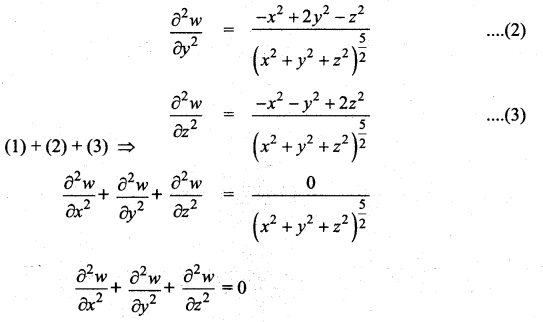Question 7.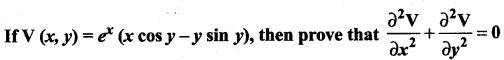Solution: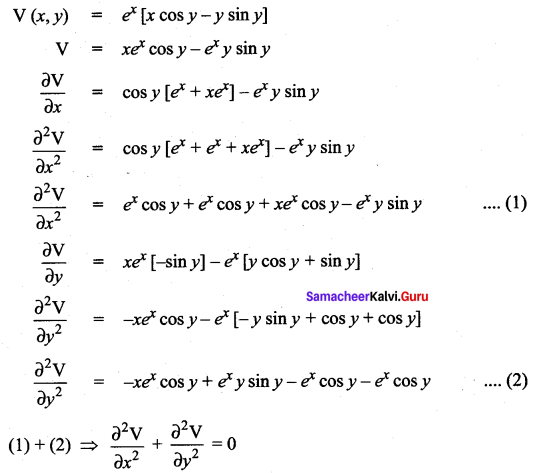Question 8.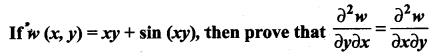Solution: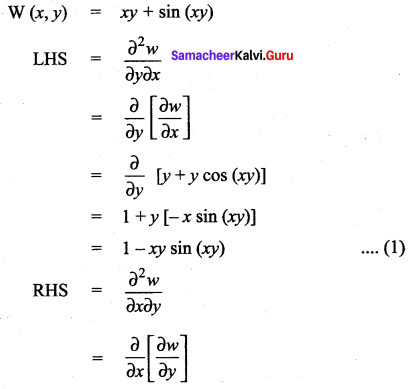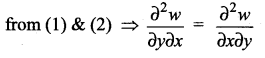Question 9.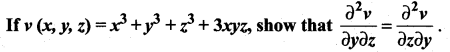Solution: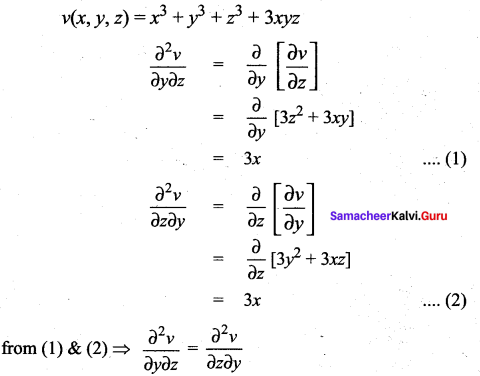Question 10.
A firm produces two types of calculators each week, x number of type A and j number of type B . The weekly revenue and cost functions (in rupees) are
R(x, y) = 80x + 90y + 0.04xy – 0.05.x2 – 0.05y2 and C(x, y) = 8x + 6y + 2000 respectively.
(i) Find the profit function P(x,y),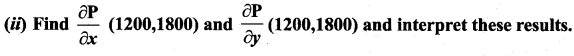Solution:
(i) Profit = Revenue – Cost
= (80x + 90y + 0.04 xy – 0.05 x2 – 0.05y2) – (8x + 6y + 2000)
= 80x + 90y + 0.04 xy – 0.05 x2 – 0.05y2 – 8x – 6y – 2000
P(x, y) = 72x + 84y + 0.04 xy – 0.05 x2 – 0.05y2 – 2000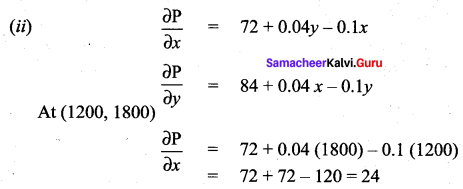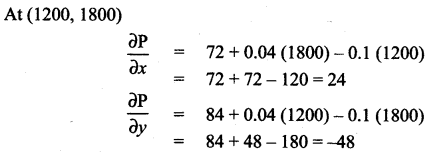### Samacheer Kalvi 12th Maths Solutions Chapter 8 Differentials and Partial Derivatives Ex 8.4 Additional Problems

Question 1.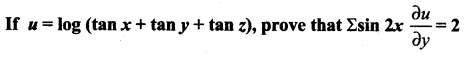Solution: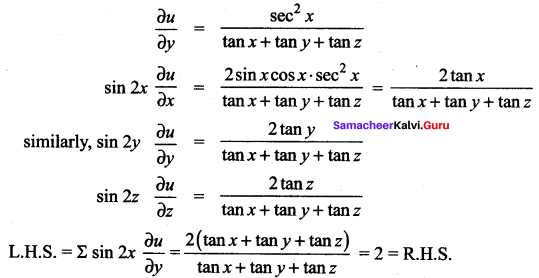Question 2.
If U = (x – y) (y – z) (z – x) then show that Ux + Uy + Uz = 0
Solution:
Ux = (y – z) {(x – y)(-1) + (z – x). 1}
= (y – z) [(z – x) – (x – y)]
Similarly Uy = (z – x) [(x – y) – (y – z)]
z = (x – y)[(y – z) – (z – x]
Ux + Uy + Uz = (y – z) [(z – x) – (z – x)] + (x – y) [- (y – z) + (y – z)] + (z – x) [(x – y) – (x – y)]
∴ Ux + Uy + Uz = 0.
Hence proved.

Question 3.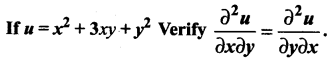Solution: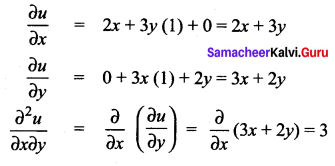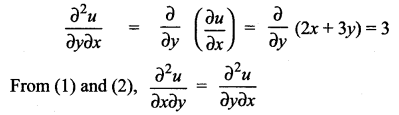Question 4.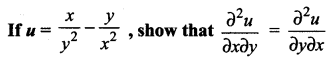Solution: